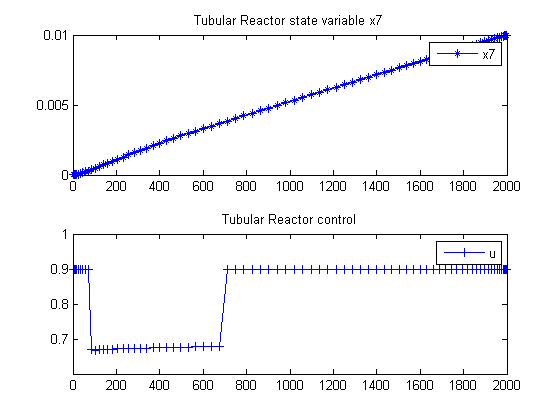118  Tubular Reactor

Global Optimization of Chemical Processes using Stochastic Algorithms 1996, Julio R. Banga, Warren D. Seider

Case Study V: Global optimization of a bifunctional catalyst blend in a tubular reactor

118.1  Problem Description

Luus et al and Luus and Bojkov consider the optimization of a tubular reactor in which methylcyclopentane is converted into benzene. The blend of two catalysts, for hydrogenation and isomerization is described by the mass fraction u of the hydrogenation catalyst. The optimal control problem is to find the catalyst blend along the length of the reactor defined using a characteristic reaction time t in the interval 0 <= t <= t_f where t_f = 2000 gh/mol corresponding to the reactor exit such that the benzene concentration at the exit of the reactor is maximized.

Find u(t) to maximize:

 J = x7(tf)

Subject to:

 dx1 dt
= −k1*x1
 dx2 dt
= k1*x1−(k2+k3)*x2+k4*x5
 dx3 dt
= k2*x2
 dx4 dt
= −k6*x4+k5*x5
 dx5 dt
= k3*x2+k6*x4−(k4+k5+k8+k9)*x5+k7*x6+k10*x7
 dx6 dt
= k8*x5k7*x6
 dx7 dt
= k9*x5k10*x7

where xi, i = 1,..,7 are the mole fractions of the chemical species (i = 1 for methylcyclopentane, i = 7 for benzene), the rate constants are functions of the catalyst blend u(t):

 ki = c(i,1)+c(i,2)*u+c(i,3)*u2+c(i,4)*u3

and the coefficients cij are given by Luus et al. The upper and lower bounds on the mass fraction of the hydrogenation catalyst are.

 0.6 <= u <= 0.9

The initial vector of mole fractions is:

 x(t0) = [1  0  0  0  0  0  0]′

Reference: 

118.2  Problem setup

p = tomPhase('p', t, 0, 2000, n);
setPhase(p);

tomStates x1 x2 x3 x4 x5 x6 x7
tomControls u

% Collocate initial guess
x0 = {icollocate({x1 == x1opt; x2 == x2opt
x3 == x3opt; x4 == x4opt; x5 == x5opt
x6 == x6opt; x7 == x7opt})
collocate(u == uopt)};

% Box constraints
cbox = {icollocate({
0 <= x1 <= 1; 0 <= x2 <= 1
0 <= x3 <= 1; 0 <= x4 <= 1
0 <= x5 <= 1; 0 <= x6 <= 1
0 <= x7 <= 1})
0.6 <= collocate(u) <= 0.9};

% Boundary constraints
cbnd = initial({x1 == 1; x2 == 0
x3 == 0; x4 == 0; x5 == 0
x6 == 0; x7 == 0});

% ODEs and path constraints
uvec = [1, u, u.^2, u.^3];
k    = (c'*uvec')';
ceq = collocate({
dot(x1) == -k(1)*x1
dot(x2) ==  k(1)*x1-(k(2)+k(3))*x2+k(4)*x5
dot(x3) ==  k(2)*x2
dot(x4) == -k(6)*x4+k(5)*x5
dot(x5) ==  k(3)*x2+k(6)*x4-(k(4)+k(5)+k(8)+k(9))*x5+...
k(7)*x6+k(10).*x7
dot(x6) == k(8)*x5-k(7)*x6
dot(x7) == k(9)*x5-k(10)*x7});

% Objective
objective = -final(x7);
options = struct;
options.name = 'Tubular Reactor';
options.d2c = false;
Prob = sym2prob('con',objective,{cbox, cbnd, ceq}, x0, options);
Prob.xInit = 35; % Use 35 random starting points.
Prob.GO.localSolver = 'snopt';
if n<=10
Result = tomRun('multiMin', Prob, 1);
else
Result = tomRun('snopt', Prob, 1);
end
solution = getSolution(Result);

% Store optimum for use in initial guess
x1opt = subs(x1,solution);
x2opt = subs(x2,solution);
x3opt = subs(x3,solution);
x4opt = subs(x4,solution);
x5opt = subs(x5,solution);
x6opt = subs(x6,solution);
x7opt = subs(x7,solution);
uopt  = subs(u,solution);
===== * * * =================================================================== * * *
TOMLAB - Tomlab Optimization Inc. Development license  999001. Valid to 2011-02-05
=====================================================================================
Problem: ---  1: Tubular Reactor - Trial 7      f_k      -0.010036498851799764
sum(|constr|)      0.000000000086184773
f(x_k) + sum(|constr|)     -0.010036498765614991

Solver: multiMin with local solver snopt.  EXIT=0.  INFORM=0.
Find local optima using multistart local search
Did 35 local tries. Found 1 global, 33 minima. TotFuncEv 1546. TotConstrEv 1477

FuncEv 1546 GradEv 1476 ConstrEv 1477 ConJacEv   37 Iter  921
CPU time: 11.156250 sec. Elapsed time: 11.485000 sec.
===== * * * =================================================================== * * *
TOMLAB - Tomlab Optimization Inc. Development license  999001. Valid to 2011-02-05
=====================================================================================
Problem: ---  1: Tubular Reactor                f_k      -0.009997531214886855
sum(|constr|)      0.000000159449045791
f(x_k) + sum(|constr|)     -0.009997371765841064
f(x_0)     -0.010036498851799764

Solver: snopt.  EXIT=0.  INFORM=1.
SNOPT 7.2-5 NLP code
Optimality conditions satisfied

FuncEv   28 GradEv   26 ConstrEv   26 ConJacEv   26 Iter   18 MinorIter  548
CPU time: 3.906250 sec. Elapsed time: 4.000000 sec.
end

118.3  Plot result

t  = collocate(t);
x7 = collocate(x7opt);
u  = collocate(uopt);

subplot(2,1,1)
plot(t,x7,'*-');
legend('x7');
title('Tubular Reactor state variable x7');

subplot(2,1,2)
plot(t,u,'+-');
legend('u');
title('Tubular Reactor control');